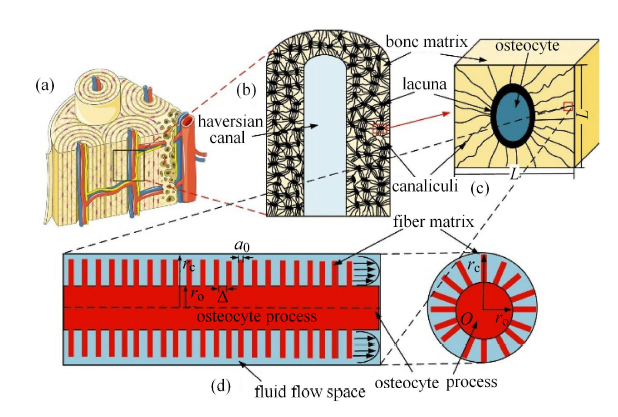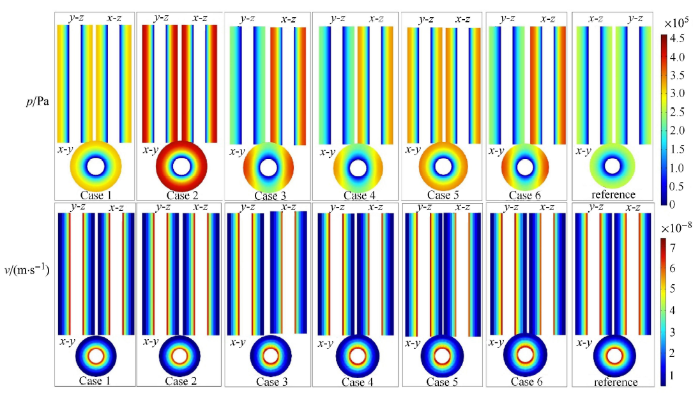## 骨陷窝-骨细胞形状和方向对骨单元内液体流动行为的影响 1)

*太原理工大学生物医学工程学院,太原 030024

## EFFECT OF OSTEOCYTE-LACUNAE SHAPE AND DIRECTION ON THE FLUID FLOW BEHAVIOR IN OSTEON 1)

Yu Weilun*, Wu Xiaogang,*,2), Li Chaoxin*, Sun Yuqin*, Zhang Meizhen, Chen Weiyi,*,3)

*College of Biomedical Engineering,Taiyuan University of Technology,Taiyuan 030024,China

College of Physical Education,Taiyuan University of Technology,Taiyuan 030024,China

 基金资助: 1)国家自然科学基金项目.  11972242国家自然科学基金项目.  11702183国家自然科学基金项目.  11572213国家自然科学基金项目.  11632013山西省高等学校科技创新项目.  2017135哲学社会科学研究项目.  2017313

Received: 2019-12-18   Accepted: 2020-02-20   Online: 2020-05-18Abstract

In order to accurately describe the fluid flow in osteon, this study developed a method to describe the fluid anisotropic flow based on the density, shape and direction of lacunae. Firstly, the number and distribution of the bone canaliculi around the lacunae were calculated. Secondly, the permeability and porosity were estimated by using the calculated parameters and other microstructure data of bone tissue. Finally, the poroelastic finite element model of osteon was established according to the calculated parameters, and the influence of lacunae shape and direction on the fluid flow behavior in osteon under the axial displacement load was analyzed. The results showed that the lacunae shape and direction had a significant effect on the value and distribution of fluid pressure and velocity in osteon. For the range of parameters investigated, the influence of the lacunae shape on the maximum pressure and flow velocity in the same region of different osteon models can reach 86% and 18%, respectively, and the influence of the lacunae direction on that can reach 125% and 56%, respectively. In addition, the lacunae shape and direction had a great influence on the local pressure and velocity of a single osteon (up to 62% difference in fluid pressure between regions due to the influence of the lacunae shape, and up to 58% and 50% difference in fluid pressure and flow velocity due to the influence of the lacunae direction, respectively). The model showed that the lacunae shape and direction and the three-dimensional distribution of the canaliculus can determine the degree of anisotropy fluid flow in osteon. This study help to accurately quantify the anisotropic flow behavior of interstitial fluid of bone.

Keywords： lacunae ; osteocyte ; poroelastic ; osteon ; bone canaliculus ; permeability

Yu Weilun, Wu Xiaogang, Li Chaoxin, Sun Yuqin, Zhang Meizhen, Chen Weiyi. EFFECT OF OSTEOCYTE-LACUNAE SHAPE AND DIRECTION ON THE FLUID FLOW BEHAVIOR IN OSTEON 1). Chinese Journal of Theoretical and Applied Mechanics[J], 2020, 52(3): 843-853 DOI:10.6052/0459-1879-19-357

## 1 基于骨单元微观结构计算其渗透率

### 图1Fig.1   The hierarchical structure for bone tissue

$k_{lcp} = \dfrac{2\pi n_i a^4q^3}{\gamma ^3L^2}\Bigg\{ A_1 \left[ { {I}_1 \left( {\dfrac{\gamma }{q}} \right) - q{I}_1 \left( \gamma \right)} \right] + \\ \qquad B_1 \left[ {q {K}_1 \left( \gamma \right) - {K}_1 \left( {\dfrac{\gamma }{q}} \right)} \right] + \dfrac{\gamma (q^2 - 1)}{2q} \Bigg \}$

$A_{1}$和$B_{1}$由以下方程得出

$\left. \begin{array}{ll} A_1 = \dfrac{{K}_0 \left( \gamma \right) - {K}_0 \left( {\gamma / q} \right)}{{I}_0 \left( {\gamma / q} \right){K}_0 \left( \gamma \right) - {I}_0 \left( \gamma \right){K}_0 \left( {\gamma / q} \right)} \\ B_1 = \dfrac{{I}_0 \left( \gamma \right) - {I}_0 \left( {\gamma/q} \right)}{{I}_0 \left( {\gamma/q} \right){K}_0 \left( \gamma \right) - {I}_0 \left( \gamma \right){K}_0 \left( {\gamma /q} \right)}\end{array} \right\}$

$L$表示两个骨陷窝之间的距离,也是CPUC的边长,可由以下公式得出

$L = \left( {\dfrac{V_L }{N_{Lac} }} \right)^{\tfrac 13 }$

### 图7Fig.7   The pore pressure ($p)$ and flow velocity ($v)$ distribution of osteon due to the influence of lacunae direction

## 5 结论

/

 〈〉